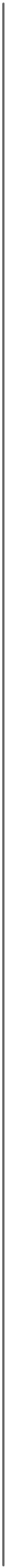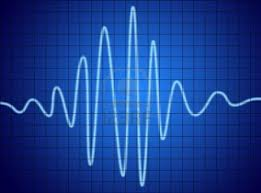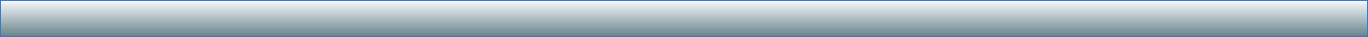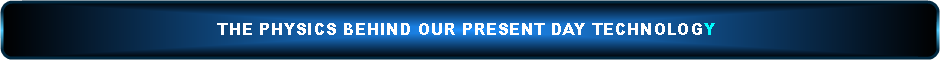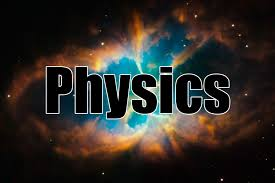High School Physics by Dan Fullerton 1
High School Physics by Dan Fullerton 2
High School Physics by Dan Fullerton 3
High School Physics by Dan Fullerton 4
Minute Physics
Michio Kaku on Physics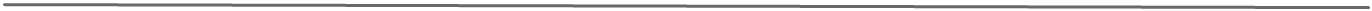MY PINTEREST PAGE ON PHYSICS
428 PINS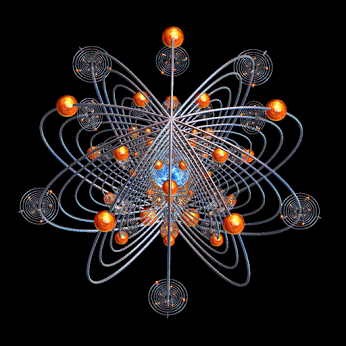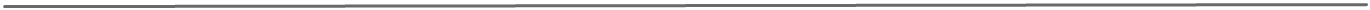WHAT IS WORK?
Work Equation

Mathematically, work can be expressed by the following equation.
W = F • d • cos Θ

where F is the force, d is the displacement, and the angle (theta) is defined as the angle between the force and the displacement vector. Perhaps the most difficult aspect of the above equation is the angle "theta." The angle is not just any 'ole angle, but rather a very specific angle. The angle measure is defined as the angle between the force and the displacement. To gather an idea of it's meaning, consider the following three scenarios.

Scenario A: A force acts rightward upon an object as it is displaced rightward. In such an instance, the force vector and the displacement vector are in the same direction. Thus, the angle between F and d is 0 degrees.

Scenario B: A force acts leftward upon an object that is displaced rightward. In such an instance, the force vector and the displacement vector are in the opposite direction. Thus, the angle between F and d is 180 degrees.

Scenario C: A force acts upward on an object as it is displaced rightward. In such an instance, the force vector and the displacement vector are at right angles to each other. Thus, the angle between F and d is 90 degrees.

When a force acts upon an object to cause a displacement of the object, it is said that work was done upon the object. There are three key ingredients to work - force, displacement, and cause. In order for a force to qualify as having done work on an object, there must be a displacement and the force must cause the displacement. There are several good examples of work that can be observed in everyday life - a horse pulling a plow through the field, a father pushing a grocery cart down the aisle of a grocery store, a freshman lifting a backpack full of books upon her shoulder, a weightlifter lifting a barbell above his head, an Olympian launching the shot-put, etc. In each case described here there is a force exerted upon an object to cause that object to be displaced.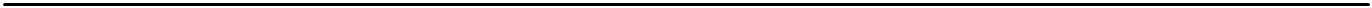TOPICS IN PHYSICS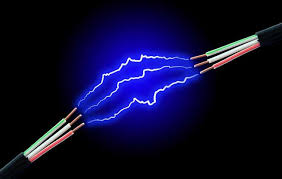To Do Work, Forces Must Cause Displacements

Let's consider Scenario C above in more detail. Scenario C involves a situation similar to the waiter who carried a tray full of meals above his head by one arm straight across the room at constant speed. It was mentioned earlier that the waiter does not do work upon the tray as he carries it across the room. The force supplied by the waiter on the tray is an upward force and the displacement of the tray is a horizontal displacement. As such, the angle between the force and the displacement is 90 degrees. If the work done by the waiter on the tray were to be calculated, then the results would be 0. Regardless of the magnitude of the force and displacement, F*d*cosine 90 degrees is 0 (since the cosine of 90 degrees is 0). A vertical force can never cause a horizontal displacement; thus, a vertical force does not do work on a horizontally displaced object!!

It can be accurately noted that the waiter's hand did push forward on the tray for a brief period of time to accelerate it from rest to a final walking speed. But once up to speed, the tray will stay in its straight-line motion at a constant speed without a forward force. And if the only force exerted upon the tray during the constant speed stage of its motion is upward, then no work is done upon the tray. Again, a vertical force does not do work on a horizontally displaced object.

The equation for work lists three variables - each variable is associated with one of the three key words mentioned in the definition of work (force, displacement, and cause). The angle theta in the equation is associated with the amount of force that causes a displacement. As mentioned in a previous unit, when a force is exerted on an object at an angle to the horizontal, only a part of the force contributes to (or causes) a horizontal displacement. Let's consider the force of a chain pulling upwards and rightwards upon Fido in order to drag Fido to the right. It is only the horizontal component of the tension force in the chain that causes Fido to be displaced to the right. The horizontal component is found by multiplying the force F by the cosine of the angle between F and d. In this sense, the cosine theta in the work equation relates to the cause factor - it selects the portion of the force that actually causes a displacement.

CONSERVATION LAW OF ENERGY
To Do Work, Forces Must Cause Displacements

Let's consider Scenario C above in more detail. Scenario C involves a situation similar to the waiter who carried a tray full of meals above his head by one arm straight across the room at constant speed. It was mentioned earlier that the waiter does not do work upon the tray as he carries it across the room. The force supplied by the waiter on the tray is an upward force and the displacement of the tray is a horizontal displacement. As such, the angle between the force and the displacement is 90 degrees. If the work done by the waiter on the tray were to be calculated, then the results would be 0. Regardless of the magnitude of the force and displacement, F*d*cosine 90 degrees is 0 (since the cosine of 90 degrees is 0). A vertical force can never cause a horizontal displacement; thus, a vertical force does not do work on a horizontally displaced object!!

It can be accurately noted that the waiter's hand did push forward on the tray for a brief period of time to accelerate it from rest to a final walking speed. But once up to speed, the tray will stay in its straight-line motion at a constant speed without a forward force. And if the only force exerted upon the tray during the constant speed stage of its motion is upward, then no work is done upon the tray. Again, a vertical force does not do work on a horizontally displaced object.

The equation for work lists three variables - each variable is associated with one of the three key words mentioned in the definition of work (force, displacement, and cause). The angle theta in the equation is associated with the amount of force that causes a displacement. As mentioned in a previous unit, when a force is exerted on an object at an angle to the horizontal, only a part of the force contributes to (or causes) a horizontal displacement. Let's consider the force of a chain pulling upwards and rightwards upon Fido in order to drag Fido to the right. It is only the horizontal component of the tension force in the chain that causes Fido to be displaced to the right. The horizontal component is found by multiplying the force F by the cosine of the angle between F and d. In this sense, the cosine theta in the work equation relates to the cause factor - it selects the portion of the force that actually causes a displacement.

Units of Work

Whenever a new quantity is introduced in physics, the standard metric units associated with that quantity are discussed. In the case of work (and also energy), the standard metric unit is the Joule (abbreviated J). One Joule is equivalent to one Newton of force causing a displacement of one meter. In other words,
The Joule is the unit of work.

1 Joule = 1 Newton * 1 meter

1 J = 1 N * m

In fact, any unit of force times any unit of displacement is equivalent to a unit of work. Some nonstandard units for work are shown below. Notice that when analyzed, each set of units is equivalent to a force unit times a displacement unit.

Non-standard Units of Work:
foot•pound kg•(m/s2)•m kg•(m2/s2)

In summary, work is done when a force acts upon an object to cause a displacement. Three quantities must be known in order to calculate the amount of work. Those three quantities are force, displacement and the angle between the force and the displacement.

Conservation of Energy

Energy can be defined as the capacity for doing work. It may exist in a variety of forms and may be transformed from one type of energy to another. However, these energy transformations are constrained by a fundamental principle, the Conservation of Energy principle. One way to state this principle is "Energy can neither be created nor destroyed". Another approach is to say that the total energy of an isolated system remains constant.
Conservation Laws

If a system does not interact with its environment in any way, then certain mechanical properties of the system cannot change. They are sometimes called "constants of the motion". These quantities are said to be "conserved" and the conservation laws which result can be considered to be the most fundamental principles of mechanics. In mechanics, examples of conserved quantities are energy, momentum, and angular momentum. The conservation laws are exact for an isolated system.

Stated here as principles of mechanics, these conservation laws have far-reaching implications as symmetries of nature which we do not see violated. They serve as a strong constraint on any theory in any branch of science
.
An Isolated System (Closed System)

An isolated system implies a collection of matter which does not interact with the rest of the universe at all - and as far as we know there are really no such systems. There is no shield against gravity, and the electromagnetic force is infinite in range. But in order to focus on basic principles, it is useful to postulate such a system to clarify the nature of physical laws. In particular, the conservation laws can be presumed to be exact when referring to an isolated system:

Conservation of Energy: the total energy of the system is constant.
Conservation of Momentum: the mass times the velocity of the center of mass is constant.
Conservation of Angular Momentum: The total angular momentum of the system is constant.
Newton's Third Law: No net force can be generated within the system since all internal forces occur in opposing pairs. The acceleration of the center of mass is zero.IN DEPTH STUDIES OF THE THERMODYNAMIC LAWS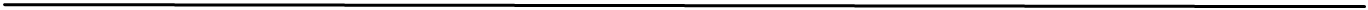MAGNETISM

Magnetic Poles, Forces, and Fields

Any object that exhibits magnetic properties is called a magnet. Every magnet has two points, or poles, where most of its strength is concentrated; these are designated as a north-seeking pole, or north pole, and a south-seeking pole, or south pole, because a suspended magnet tends to orient itself along a north-south line. Since a magnet has two poles, it is sometimes called a magnetic dipole, being analogous to an electric dipole, composed of two opposite charges. The like poles of different magnets repel each other, and the unlike poles attract each other.

One remarkable property of magnets is that whenever a magnet is broken, a north pole will appear at one of the broken faces and a south pole at the other, such that each piece has its own north and south poles. It is impossible to isolate a single magnetic pole, regardless of how many times a magnet is broken or how small the fragments become. (The theoretical question as to the possible existence in any state of a single magnetic pole, called a monopole, is still considered open by physicists; experiments to date have failed to detect one.)

From his study of magnetism, C. A. Coulomb in the 18th cent. found that the magnetic forces between two poles followed an inverse-square law of the same form as that describing the forces between electric charges. The law states that the force of attraction or repulsion between two magnetic poles is directly proportional to the product of the strengths of the poles and inversely proportional to the square of the distance between them.

As with electric charges, the effect of this magnetic force acting at a distance is expressed in terms of a field of force. A magnetic pole sets up a field in the space around it that exerts a force on magnetic materials. The field can be visualized in terms of lines of induction (similar to the lines of force of an electric field). These imaginary lines indicate the direction of the field in a given region. By convention they originate at the north pole of a magnet and form loops that end at the south pole either of the same magnet or of some other nearby magnet (see also flux, magnetic). The lines are spaced so that the number per unit area is proportional to the field strength in a given area. Thus, the lines converge near the poles, where the field is strong, and spread out as their distance from the poles increases.

A picture of these lines of induction can be made by sprinkling iron filings on a piece of paper placed over a magnet. The individual pieces of iron become magnetized by entering a magnetic field, i.e., they act like tiny magnets, lining themselves up along the lines of induction. By using variously shaped magnets and various combinations of more than one magnet, representations of the field in these different situations can be obtained.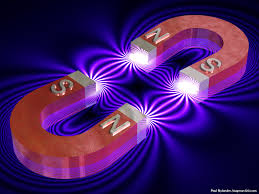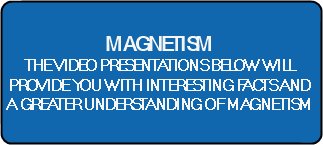YOUR SOURCE FOR PEAK PERFORMANCE MAGNETSA GREAT TUTORIAL ON MAGNETISM FROM EXPLAINTHATSTUFF.COM
Quantum mechanics (QM; also known as quantum physics or quantum theory), including quantum field theory, is a fundamental branch of physics concerned with processes involving, for example, atoms and photons. Systems such as these which obey quantum mechanics can be in a quantum superposition of different states, unlike in classical physics.

Quantum mechanics gradually arose from Max Planck's solution in 1900 to the black-body radiation problem (reported 1859) and Albert Einstein's 1905 paper which offered a quantum-based theory to explain the photoelectric effect (reported 1887). Early quantum theory was profoundly reconceived in the mid-1920s.

The reconceived theory is formulated in various specially developed mathematical formalisms. In one of them, a mathematical function, the wave function, provides information about the probability amplitude of position, momentum, and other physical properties of a particle.

Important applications of quantum theory include superconducting magnets, light-emitting diodes and the laser, the transistor and semiconductors such as the microprocessor, medical and research imaging such as magnetic resonance imaging and electron microscopy, and explanations for many biological and physical phenomena.Animation explaing quantum physics
SECRETS OF QUANTUM PHYSICS
MY PINTEREST PAGE ON QUANTUM MECHANICS
This work has been released into the public domain by its author, PoorLeno at English Wikipedia.
376 PINS ON QUANTUM MECHANICS AND QUANTUM PHYSICS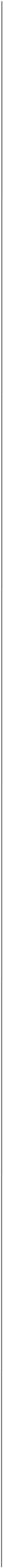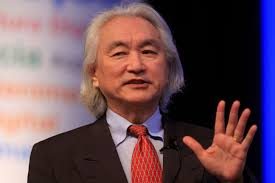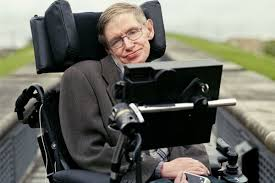LOCKHEEDS FUSION REACTOR
SCIENTISTS DEVELOP A LASER VERSION OF MAXWELLS DEMON TO GET CLOSER TO QUANTUM COMPUTING
LAWS OF MAGNETISM AND ELECTRICITY
This is a non-profit web site, dedicated to educational study and research. All copyrights belong to their owners and are acknowledged. All material presented on this web site is either news reporting or information presented for non-profit study and research, or has previously been publicly disclosed or has implicitly or explicitly been put into the public domain. Fair Use applies.